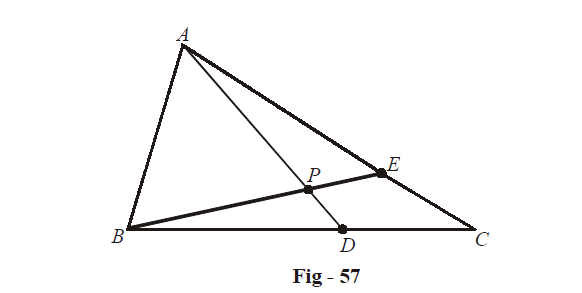# Examples On Vector Equations Of Lines Set-1

Go back to  'Vectors and 3-D Geometry'

Learn from the best math teachers and top your exams

• Live one on one classroom and doubt clearing
• Practice worksheets in and after class for conceptual clarity
• Personalized curriculum to keep up with school

Example – 44

In  $$\Delta ABC,$$ D and E are points on BC and AC respectively such that $$BD = {\text{ }}2DC\,\,and\,\,AE = {\text{ }}3EC$$. Let P be the point of intersection of AD and BE. Find using vector methods.

Solution:There’s no loss of generality in assuming A to be the origin $$\vec 0,$$ and B and C to be the points $$\vec b$$ and $$\vec c$$ respectively. By the section formula,

$D \equiv \frac{{b + 2\vec c}}{3},\,E \equiv \frac{{3\vec c}}{4}$

The equation of AD can be written (in parametric form) as:

\begin{align}&\qquad\quad \vec r = \vec 0 + \lambda \left( {\frac{{\vec b + 2\vec c}}{3}} \right)\qquad\qquad\qquad\qquad,\lambda \in \mathbb{R} \hfill \\\\ & \Rightarrow \quad \vec r = \left( {\frac{\lambda }{3}} \right)\vec b + \left( {\frac{{2\lambda }}{3}} \right)\vec c \hfill \\ \end{align}

Similarly, the equation of BE can be written as

\begin{align}&\;\; \qquad\vec{r}=\vec{b}+{\lambda }'\left( \frac{3\vec{c}}{4}-\vec{b} \right)\qquad\qquad\qquad\qquad,{\lambda }'\in \mathbb{R} \\\\ & \Rightarrow \quad \vec{r}=\left( 1-{\lambda }' \right)\vec{b}+\left( \frac{3{\lambda }'}{4} \right)\vec{c} \\ \end{align}

AD and BE intersect at P. Thus, the position vector of P must satisfy the equations of both AD and BE. This means that we must have

\begin{align}& \left( \frac{\lambda }{3} \right)\vec{b}+\left( \frac{2\lambda }{3} \right)\vec{c}=\left( 1-{\lambda }' \right)\vec{b}+\left( \frac{3{\lambda }'}{4} \right)\vec{c}\,\,for\text{ }some\,\,\lambda ,\,{\lambda }'\in \mathbb{R} \\\\ & \Rightarrow \quad\left( \frac{\lambda }{3}+{\lambda }'-1 \right)\vec{b}+\left( \frac{2\lambda }{3}-\frac{3{\lambda }'}{4} \right)\vec{c}=\vec{0} \\ \end{align}

Since $$\vec{b}\;\,and\;\,\vec{c}$$ are non-collinear, we must have

\begin{align} & \frac{\lambda }{3}+{\lambda }'-1=0 \\\\ & \frac{2\lambda }{3}-\frac{3{\lambda }'}{4}=0 \\ \end{align}

This system upon solving yields

$\lambda =\frac{9}{11},\,\,{\lambda }'=\frac{8}{11}$

Thus, the position vector of P can be obtained by substituting the value of $$\lambda (\text{or }{\lambda }')$$ in the equations for AD (or BE):

$P\equiv \frac{3}{11}\left( \vec{b}+2\vec{c} \right)$

We now know the position vectors of B, P and E. We simply need to find $$BP:PE.$$ Suppose this is $$m:1.$$ Then,

\begin{align}&\qquad \frac{m\left( \frac{3\vec{c}}{4} \right)+1\left( {\vec{b}} \right)}{m+1}=\frac{3}{11}\left( \vec{b}+2\vec{c} \right) \\\\ & \Rightarrow \quad33m\vec{c}+44\vec{b}=12\left( m+1 \right)\vec{b}+24\left( m+1 \right)\vec{c} \\\\ & \Rightarrow \quad\left( 32-12m \right)\vec{b}+\left( 9m-24 \right)\vec{c}=\vec{0} \\\\ & \Rightarrow \quad m=\frac{8}{3} \\ \end{align}

Thus,

$BP:PE=8:3$

The technique used in this example, in particular the evaluation of P as the intersection point of two lines (whose lines are in vector form), is very important and useful and you must understand it properly before proceeding.

Let us see more examples of this sort.

Example – 45

Let $$\vec{a}=\hat{i}+\hat{j}\,\,and\,\,\vec{b}=2\hat{i}-\hat{k}.$$ Find the point of intersection of the lines  $$\vec{r}\times \vec{a}=\vec{b}\times \vec{a}\;\,and\,\;\vec{r}\times \vec{b}=\vec{a}\times \vec{b}.$$

Solution: Both the lines have been specified in non-parametric form, which we can easily convert to parametric form.

\begin{align} & \vec{r}\times \vec{a}=\vec{b}\times \vec{a}\Rightarrow \quad \vec{r}=\vec{b}+\lambda \vec{a}\;\;\qquad\qquad where\;\;\;\lambda \in \mathbb{R} \\\\ & \vec{r}\times \vec{b}=\vec{a}\times \vec{b}\Rightarrow \quad\vec{r}=\vec{a}+{\lambda }'\vec{b}\;\;\qquad\qquad where\;\;\;{\lambda }'\in \mathbb{R} \\ \end{align}

If these two lines intersect, then we must have some values of $$\lambda ,\,{\lambda }',$$ say $${{\lambda }_{0}}\,and\,{{{\lambda }'}_{0}},$$ such that

\begin{align}&\qquad\quad \vec{b}+{{\lambda }_{0}}\vec{a}=\vec{a}+{{{{\lambda }'}}_{0}}\vec{b} \\\\ & \Rightarrow\quad \left( 1-{{{{\lambda }'}}_{0}} \right)\vec{b}+\left( {{\lambda }_{0}}-1 \right)\vec{a}=\vec{0} \\ \end{align}

Since  $$\vec{a}\,\,and\,\,\vec{b}$$ are non-collinear, we must have $${{\lambda }_{0}}={{{\lambda }'}_{0}}=1.$$ The position vector of the point of intersection P can now be evaluated by substituting $${{\lambda }_{0}}\,\;or\;\,{{{\lambda }'}_{0}}$$ in the corresponding equation:

\begin{align}&\qquad\quad P\equiv \vec{b}+\vec{a}=\vec{a}+\vec{b} \\\\ &\;\; \Rightarrow\quad P\equiv 3\hat{i}+\hat{j}-\hat{k} \\ \end{align}

Vectors
grade 11 | Questions Set 1
Vectors
Vectors
grade 11 | Questions Set 2
Vectors
Three Dimensional Geometry
grade 11 | Questions Set 2
Three Dimensional Geometry
Three Dimensional Geometry
grade 11 | Questions Set 1
Three Dimensional Geometry
Vectors
grade 11 | Questions Set 1
Vectors
Vectors
grade 11 | Questions Set 2
Vectors